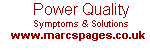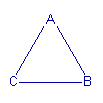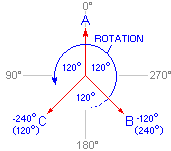CAUSES:Phase Rotation  I just love standards. Just when you thought things were logical, someone came along and created an opposite standard. Now, does this mean the first is obsolete? No. Not until the generation who learned the first standard are now dead and buried - but then comes those that they taught! Phase rotation has this very problem in that what defines "clockwise" and "anti-clockwise".If one looks at a usual picture of a 3-phase connection, it is not unusual to find it represented as an equilateral triangle with phase A as the apex, and B & C as the two base points with A, B, & C ordered in a clockwise manner. It is accepted, in the "conventional" connection, that A is the reference and that everything relates to it. As waveforms are based on cyclic behaviour, degrees are important and A is given the reference of 0°. This would place B at 120° and C at 240° with respect to A. If coils were placed around a compass at 120° spacing with each connected to the respective phase at that point, the compass would first be attracted to A, then B, and finally C before returning to A. This clearly indicates a clockwise rotation.For metering purposes one needs to know how accurately the phases are divided over time. The known method is to represent a complete cycle on A as a circle, and to then indicate where phases B and C reach their 0° point on A's cycle. This, in a well balanced feed, would show B reaching 0° when A is at 120°, and C reaching 0° with A at 240°. Now forget everything we have just said because there is another argument. Lets replace our compass with an electric motor. The same result is the shaft of the motor turns clockwise (are we going down the same track here?). The problem is the argument says the torque on the motor is anti-clockwise (the motor has a tendency to want to turn and if it was not for the fact it was fixed...... it would!).This argument is founded on the principle of ascertaining which direction the phases have to turn for each to go through the 0° point in the correct order (the direction the coils need to turn in order to keep the compass needle facing 0°). If one studies the accompanying picture one will see we took a 'snapshot' as A went through 0°. For B to reach 0° the 'propeller' has to rotate...... anti-clockwise! In the above 'snapshot' the angles of the phases are now given as "where B & C are at on their respective cycles as A goes through 0°". This would put B at 240° and C at 120°. To substantiate this argument, one needs to think of degrees as time. In this line of thought it would mean B lags behind A by 120° (it must still complete 120° of rotation before it reaches the zero point i.e. 0°), and C 120° behind B (making C lag 240° behind A). B is therefore at -120° and C -240°. As there are 360° in a circle, B's position could also be shown as -120+360=240°, C as 120°. Yes, we have just proved that time stands still and that we are moving through time (or is it that we stand still and time moves? - Oh! whatever!). You can probably tell, I'm of the school that believes that a conventional connection with A peaking first, followed by B then C, is clockwise rotation. But is there a difference.... ? The description of clockwise rotation is distinctly based on the magnetic compass i.e. 90° is shown towards the right or East, and the rotation is based on where the phases have created a magnetic north using coils spread 120° apart. The electrical compass is based on what some call the "mathematical compass" i.e. 90° is towards the left, and this is, strangely, an exact mirror of the magnetic compass (have a look above). If we were to mirror either the clockwise or anti-clockwise description (such that both compass angles were to align), one finds that both descriptions are representative of each other i.e. they are exactly the same thing! What is different is the angle of view i.e. it's the difference between viewing the direction of spin of a propeller on a plane, or holding on to the propeller and seeing which way the plane spins. The real question then is which view is more correct? Well, the answer is.... neither! However, Because electrical energy is not always converted into rotating magnetic energy, the magnetic compass is less used than the mathematical thus it has become industry standard to define the rotation of the sequence of A-B-C as anti-clockwise (as illogical and unnatural as this may seem). There are extreme dangers in this because one simply has to start looking at current phase angle with leading power factor being regarded as 'negative' i.e. as though it were the same direction as the B-phase (being -120°). But we all know the B-phase lags the A-phase so using a negative notation to indicate a leading power factor is kind of crackers; Yet that is what's done! It clearly shows it was time for a new standard, one that works on sequence rather than some compass (it is covered later in the section on 'Imbalance'). The problem exists with knowing to which 'standard' the instrumentation complies with. Remember there is no right and wrong, just simply two modes of thinking. Changing Colours  >>| | Ask a Question | © 13.07.01 / 12.07.06